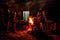# Neural network pruning with simulated annealingPhoto by Ludomił on Unsplash

The flexibility and adaptability of neural networks make them a great tool for several machine learning applications. However, neural networks and deep neural networks some times need a huge amount of resources to make the inference. Currently, new techniques are being developed to make smaller networks as well as to make the evaluation a little bit faster. One of those techniques is called pruning, an operation performed in neural networks to eliminate all the weights that add little to no information to the final output. In this post, I will show you how to minimize the number of weights used in a neural network.

# Predicting the time series.

First, we train a deep neural network on a time series data. The data consists of DLS/EUR exchange prices from about a year ago. Unfortunately, I cannot post the data set but the concept can be extended to another time series and neural network.

Once we loaded the data, we preprocess the data by applying a statistical normalization with scikit -learn with:

Once the data is normalized, we use a sliding window to create two data sets. The first one consists of a fragment of n consecutive values, which will be the training data. The second data set consists of the n+1 value from the time series, which will be the target of the neural network.

Know that we have the data prepared for training, we train a five-layer neural network with the current architecture:

With the neural network trained we can start to analyze the weights of each layer.

We can access the weights of each layer with:

We can find that the weights had a resemblance to a normal distribution on each layer. Also, the mean value for the weights is around zero, meaning that some weights have little impact on the outcome.

To test the previous hypothesis we can randomly trim weights in the network and evaluate the mean squared error as a measure of network performance.

From that approach, we can find that by removing a percentage of the total weights in the network, at low percentage values there is no change in the network performance and by increasing the number of removed weights there is no clear trend, as we increase the trimming percentage, there are some values where the performance seems to be similar to the original network. Meaning that some specific weights in the network are critical for the final prediction.

# Pruning the network

We can see the neural network pruning process as a resource allocation problem, where we want to eliminate weights that add little information to the final output. We input a list of integer values that correspond to the location of the weights to be trimmed and the output will be a list with the trimmed weights. To optimize the list of integer values we are going to use the simulated annealing algorithm.

The simulated annealing algorithm is a metaheuristic algorithm that can be described in three basic steps. First, a random initial state is created and we calculate the energy of the system or performance, then for k-steps, we select a neighbor near the current state and calculate the energy in the new state. Finally, we select the state as a feasible solution if the probability evaluated at the current state, the previous state and the current temperature (k/k-steps) is greater than a randomly generated threshold between 0 and 1. As the algorithm iterates through the solution space, the probability to accept a bad solution decreases, this decrease is similar to the controlled cooling used in metallurgic processes.

In our current application, the neighbor state is generated by randomly selecting values in the integer list, and then adding a random value to that integer.

By applying simulated annealing to the weights list we can trim about 5% of the weights in the network with a small decrease in the network performance.

Know you’re able to process the data and train a neural network to predict time series. And how to trim the weights in a neural network with simulated annealing. The complete code for this tutorial can be found in my GitHub by clicking here. See you in the next one.

Writing about math, natural sciences and academia.

## More from Octavio Gonzalez-Lugo

Writing about math, natural sciences and academia.